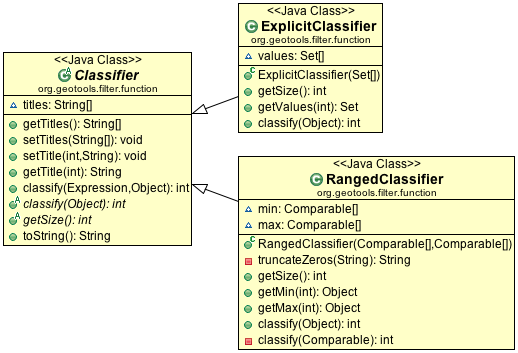# Classifier¶

A classifier captures a group of values (either expressed explicitly or as a range) providing a title to each group.You can consider a Classifier as a set of groups into which you can sort your data:

• ExplicitClassifier

Definition of explicit classifier by hand:

```        Set[] zones = new Set;
// urban commercial or residencial
zones =
new HashSet(
Arrays.asList(
new String[] {
"Zone 1", "Zone 2",
}));
// municipal or crown parkland
zones =
new HashSet(
Arrays.asList(
new String[] {
"Zone 4", "Crown 2",
}));
// industrial
zones = new HashSet(Arrays.asList(new String[] {"Zone 3"}));

Classifier landuse = new ExplicitClassifier(zones);
landuse.setTitle(0, "urban");
landuse.setTitle(1, "park");
landuse.setTitle(2, "industrial");
```
• RangedClassifier

Definition of a ranged classifier by hand:

```        Comparable min[] = new Comparable;
Comparable max[] = new Comparable;
for (int i = 0; i < 25; i++) {
min[i] = (char) ('A' + i);
max[i] = (char) ('B' + i);
}
Classifier alphabetical = new RangedClassifier(min, max);
```
• As shown above each group can be provided with a title; these titles will be used in the generated styles:

```        groups.setTitle(0, "Group A");
groups.setTitle(1, "Group B");
```
• You can also automatically generate a Classifier from a FeatureCollection.

The following “classifier” functions are available:

• EqualInterval - classifier where each group represents the same sized range

• Jenks - generate the Jenks’ Natural Breaks classification

• Quantile - classifier with an even number of items in each group

• StandardDeviation - generated using the standard deviation method

• UniqueInterval - variation of EqualInterval that takes into account unique values

Here is the code example with quantile:

```        FilterFactory2 ff = CommonFactoryFinder.getFilterFactory2();
Function classify = ff.function("Quantile", ff.property("name"), ff.literal(2));

Classifier groups = (Classifier) classify.evaluate(collection);
```

Example using EqualInterval to break down a continuous range of values (such as height):

```        FilterFactory2 ff = CommonFactoryFinder.getFilterFactory2();
Function classify = ff.function("EqualInterval", ff.property("height"), ff.literal(5));

// this will create a nice smooth series of intervals suitable for presentation
// with:
// - sequential color palette to make each height blend smoothly into the next
// - diverging color palettes if you want to make higher and lower areas stand out more
Classifier height = (Classifier) classify.evaluate(collection);
```

Example using Quantile to break up a list of items (such as zones):

```        FilterFactory2 ff = CommonFactoryFinder.getFilterFactory2();
Function classify = ff.function("Quantile", ff.property("zone"), ff.literal(2));

// Zones assigned by a municipal board do not have an intrinsic numerical
// meaning making them suitable for display using:
// - qualitative palette where each zone would have the same visual impact
Classifier groups = (Classifier) classify.evaluate(collection);
```